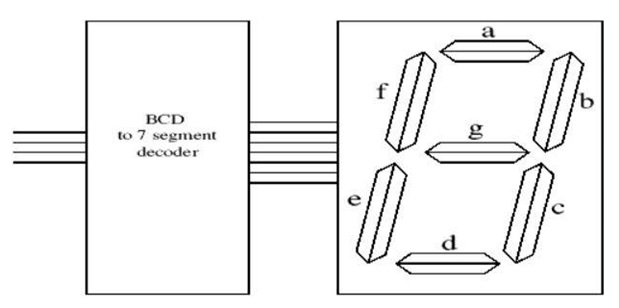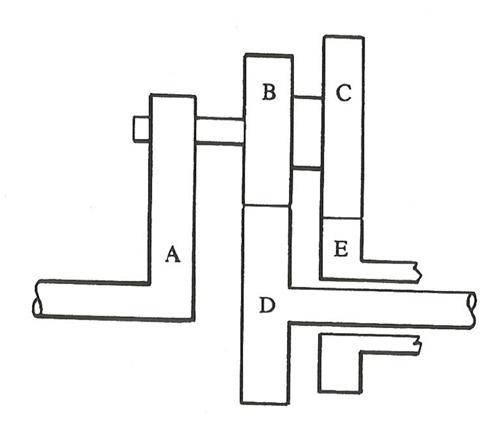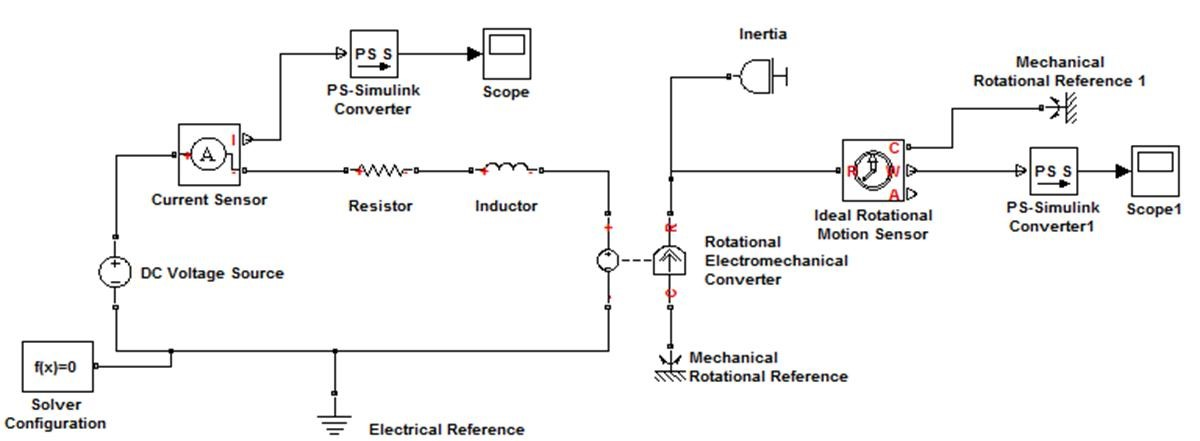# ENGT5101 Electromechanics

Section A - Analogue Electronics

A1.

1. Using diagrams, explain how an “H-Bridge” circuit can be used to control the direction of rotation and rotational speed of a d.c. motor.

(12 marks)

b)

1. With the aid of a diagram, show how a resistor and a capacitor can be used to implement a low-pass filter.

(3 marks)

1. If the resistor has a value of 10kΩ, calculate the value of capacitor required to give a critical frequency of 500Hz. Suggest the nearest “preferred value” of capacitor.

(5 marks)

A2.

1. If a d.c. motor is rated at “24 Volts, 60 Watts”, draw the circuit of a suitable mains-operated power supply and calculate the voltage and current ratings of an appropriate transformer.

(11 marks)

1. Control and transducer signals often need “conditioning” in order to correctly interface to systems. With the aid of a diagram, briefly explain how you would:
1. Reduce the amplitude of a +10 Volts d.c. signal to +5 Volts d.c. signal.

(4 marks)

1. Modify a sinewave with an amplitude range of +2 to -2 Volts into a sinewave with an amplitude range of 0 to 4 Volts.

(5 marks)

Section B - Digital Electronics

B1.

1. Seven segment displays are available in two types: common cathode, and common anode. With the aid of diagrams, distinguish between these types and explain what effect each has in terms of circuit design.

(2 marks)

1. Figure B1 shows a BCD to 7 segment decoder. You are required to design a part of this decoder – a circuit to drive segment f You should assume that the display is of the common cathode type.Figure B1

1. Develop a truth table which describes the operation of the complete (all segments) decoder.

(3 marks)

1. Derive a canonical sum of products expression from the truth table for segment f

(3 marks)

• Sketch a circuit based upon the canonical sum of products. (3 marks)
1. Produce a Karnaugh map for segment f.

(3 marks)

1. Derive a minimal sum of products expression from the Karnaugh maps.

(3 marks)

1. Show how a NAND network may be used to implement the minimal sum of products expression.

(3 marks)

B2.

1. With the aid of diagrams, explain why Gray codes are superior to natural binary in rotary encoders. Use two bit codes to illustrate your argument.

(3 marks)

1. Generate a 4 bit (i.e. 16 groups) Gray code sequence.

(3 marks)

1. From this code sequence, extract 10 groups which maintain the Gray code property.

(3 marks)

1. Using four D type flip-flops, design a 'counter' which ‘counts’ in the sequence derived in c) above.

(8 marks)

1. Comment on any initialisation requirements of your design.

(3 marks)

Section C - Mechanics

C1.

1. Determine the energy stored in a flywheel which has a moment of inertia of 0.4kgm2 and is rotating at 800rev/min. Estimate the braking torques necessary to bring the flywheel to rest in 20seconds.

(5 marks)

1. List three advantages of the V-belt drive over the flat belt drive. (3 marks)
1. A multiple V-belt drive is required to transmit 20kW.

The smaller pulley has a diameter of 160mm, an angle of lap of 165° and rotates at 1500rev/min.

The V-grooves has angles of 50° and the coefficient of friction between the belt and pulley is 0.3.

The belt material has a mass of 0.4kg/m.

If the tension in a belt is restricted to 800N, determine the number of belts required.

(12 marks)

C2.

1. Describe in detail, with appropriate sketches and relevant mathematical equations, the three main factors that need to be taken into account when selecting a motor for rotating the load with a gear transmission.

(8 marks)

1. Two co-axials shafts A and B are connected by a single plate friction clutch of internal diameter 100mm and external diameter 200mm, both sides of the plate being used.

Shaft A is rotating at a constant angular speed of 250rev/min and B is initially stationary.

Shaft B has an effective moment of inertia of 11kgm2.

When the clutch is engaged, it takes 5s for B to reach full speed.

If the coefficient of friction is 0.3 and the uniform pressure condition can be assumed, determine the required spring force and pressure.

(12 marks)

C3.

1. Describe the meaning of ‘mechanical advantage’ (MA) in engineering, and provide the definition of MA in terms of forces. List four classical simple machines used to achieve MA.

(4 marks)

1. By sketching a block on an inclined plane, and by resolving the forces acting on the block, prove that the Friction Angle, θ = tan−1µ, where µ is the coefficient of friction.

(4 marks)

1. The epicyclical gear train shown in Figure C3 has shaft A rotating at 100 rev/s.
• Wheel B has 30 teeth and Wheel C 36 teeth
• Wheel B meshing with wheel D which has 24 teeth and is keyed to the output shaft
• Wheel C meshing with the fixed wheel E
• Wheels B and C are fixed together

By constructing a table of motion for the gears, determine the angular speed of the output shaft D.Figure C3. An Epicyclical Gear Train

(12 marks)

C4.

1. Define the term Model-Based Design.

Based on the Simscape Model of a DC Motor as shown in Figure C4, briefly describe the function of the Solver Configuration, PS-Simulink Converter, Mechanical Rotational Reference and Inertia blocks.

Name two common Rotational Motion Sensors that can be used and briefly describe their operation.Figure C4. A DC Motor Model

(10 marks)

1. A mass of 3.0kg is suspended from a vertical spring of stiffness 80N/m. When the mass is displaced and released it is found that two successive amplitudes of the oscillation are 200mm and 90mm. Determine the frequency of undamped motion, the damping ratio, the damping coefficient and damped natural frequency.

(10 marks)